# A. Vector and Tensor Notation

FOR THE SAKE of convenience and to better describe the structure of the moment equations, a few symbols will be introduced. Let,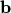,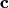,be vectors in a (real) space of dimension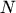.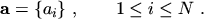(A.1)

Then, the symbol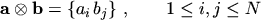(A.2)

represents the set of all pairs of the components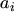,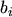of two vectors, where the indicesand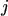range independently over all the admissible values. Hence, eqn. (A.2) defines a tensor, whose elements are the quantities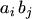. The definition can be extended to the triplets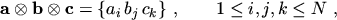(A.3)

and so on. The following notation is adopted :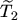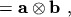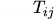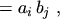(A.4)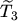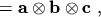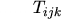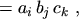(A.5)

where the numerical subscript refers to the rank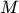of the tensor. The rank equals the number of vectors defining it. For instance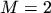in eqn. (A.2) and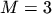in eqn. (A.3). Hence the tensor has in general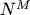different elements. From eqn. (A.2) it follows, that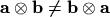. In the special case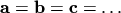, however, the tensor is completely symmetric and any permutation of indices in an element leaves the tensor unchanged. In this case, the number of elements which are different from each other is smaller than, and the tensor is invariant when the order of the vectors is changed.

It is useful to expand the tensor product to scalars as well, and to identify it with the normal product,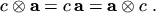(A.6)

In the following, the scalar product will be used as well. If necessary for the sake of clarity, it will be expressed explicitly like, for example in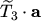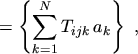(A.7)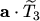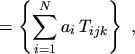(A.8)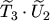(A.9)

In general, the scalar product depends on the order of the tensor, and becomes invariant only when all tensors involved are symmetric.

Let now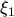,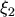,,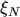be spatial coordinates, which will also be referred to as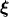, and let the tensors be functions ofas well. Introducing the nabla operator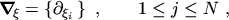(A.10)

the divergence of a ranktensor is defined as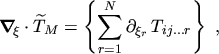(A.11)

where it is intended, that the multiplication by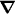is symbolic. It is seen, that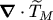is a tensor of rank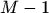. Consistently, the gradient of a ranktensor is defined as the rank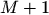tensor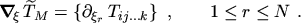(A.12)

From eqn. (A.11) and eqn. (A.12) useful identities can be derived. For any, the following relationships hold: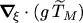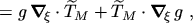(A.13)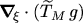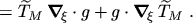(A.14)

Another definition which will be used is the statistical average of a tensor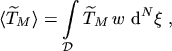(A.15)

where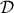is the definition domain of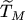in the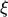-space and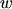is a distribution function.

M. Gritsch: Numerical Modeling of Silicon-on-Insulator MOSFETs PDF